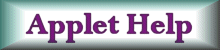Time evolution of a radioactive sample. Topics:  Radioactive decay, radioactivity, exponential rate.Pre-requisite skills:  Knowledge of the exponential function. Approximate completion time:  Under an hour. Provide sufficient detail to verify that the assignment was completed in a meaningful manner.Applet by Fu-Kwun Hwang The above applet originally displays 1950 black circles, each representing a radioactive isotope.  The half life is entered in the text box.    These black isotopes will decay into green isotopes, which are not shown in the applet window.1.  Check the box on the far right of the applet.  This will create a two-step decay process (black to white).  Set the value for tau1 = 10 (the half-life of the black isotopes) and run the applet.    The black line plots the number of black isotopes remaining (the red line has no meaning in this part of the assignment). . (a)  What percentage of the black isotopes should have decayed after 10 seconds? (b) The first horizontal tic represents 10 seconds in this part of the assignment.   Using the mouse, place the cursor on the black line directly above the first horizontal tic mark.  According to the display, how many black isotopes remain?    How does this value compare to the value predicted in Question 1(a). 2. Remove the check in the small box at the upper-right of the applet.   Also, set tau3 and tau4 equal to 0.  This will turn the radioactive series into a black, to red, to blue sequence. (a) Run the applet.  Which of the isotopes, (black, red, or blue) is the stable isotope in this simulation? (b)  The red line plots the number of red isotopes appearing at any time.   The blue line serves an analagous purpose for the blue isotopes.  Explain why the red line exhibits a peak, whereas the black and blue lines do not.  (Hint:   In order for a red isotope to be created, a black isotope must exist.) 3.  The relationship between the number of isotopes remaining after a time t is given by N = N0 Exp[-t/T], where N0 is the original number of isotopes and T is a constant that determines the rate of decay. (a)  Since there were originally 1950 black isotopes at the start of the simulation, run the applet to determine the value of the constant T (called the "median time constant"). (b)  What is the mathematical relationship between the median time constant T and the half-life, tau?   Is the median time constant T smaller or larger than the half-life? Helpful Resources Isotopes by Physics 2000. Table of the Nuclides by Jonghwa Chong Chart of the Nuclides by Jun-ichi Katakura Tables of Nuclear Data by Nuclear Data Center Natural Radioactive Series by Yevgeniy Miretskiy Radioactive Decay by Angel Franco Garcia Law of Radioactive Decay by Walter Fendt Radioactivity Unit Converter by Department of Environmental Health and Safety Return to Web Assignments